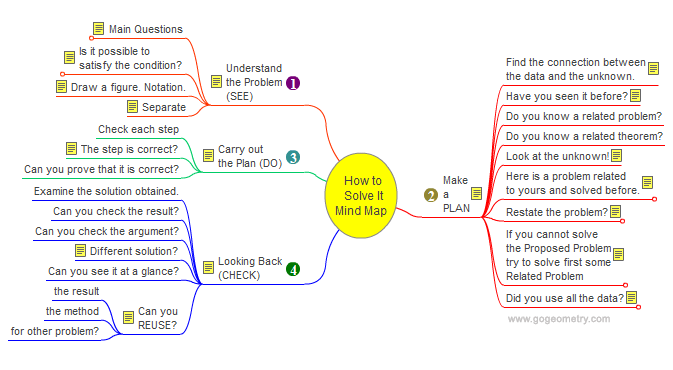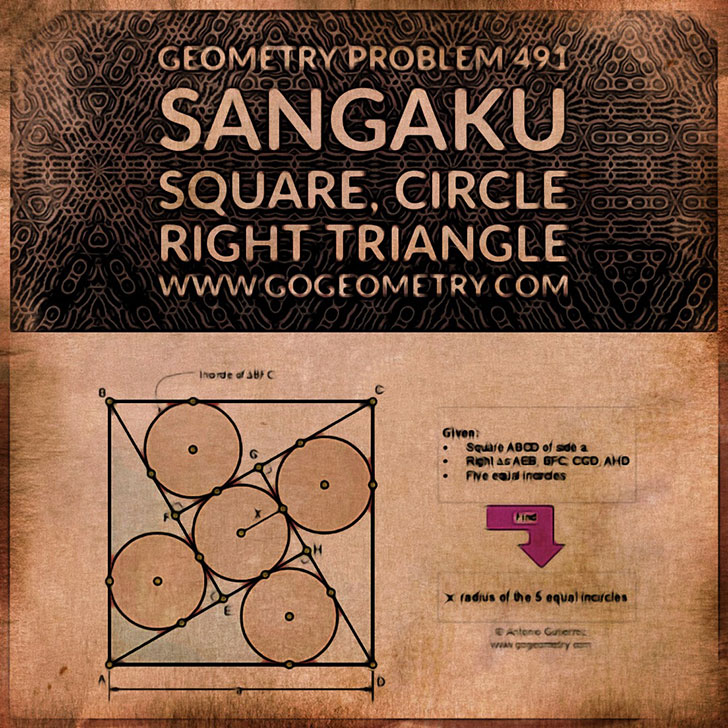Problem 491. Sangaku, Square, Right Triangle, Incircle, Inscribed Circle, Radius. Level: High School, College. The figure shows a square ABCD of side a with the right triangles AEB, BFC, CGD, and AHD. If the incircles of AEB, BFC, CGD, AHD, and EFGH are equal, find the radius x. Incircle or Inscribed circle of a polygon is a circle tangent to each side of the polygon.How to Solve It, Interactive Mind Map George Pólya's 1945 book "How to Solve It, A new aspect of Mathematical Method", is a book describing methods of problem solving. It suggests the following steps when solving a mathematical problem: (1) First, you have to understand the problem. (2) After understanding, then make a plan. (3) Carry out the plan. (4) Look back on your work. How could it be better?Moku hanga or Japanese Woodblock printing of problem 491Recent Additions